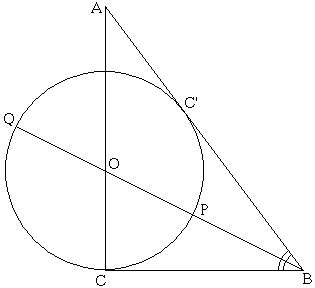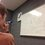# The Golden Ratio: 3-4-5Here is the previous post concerning the Golden Ratio. For a collection of all the posts concerning the Golden Ratio, click #GoldenRatio below.

Since we have already talked about several Golden figures, we are going to delve more deeply into where exactly we can find the golden ratio in other figures. Today we will talk about the ubiquitous $3-4-5$ triangle. Everyone has learned that this is the simplest of all integral right triangles. But interestingly enough, it also contains the golden ratio. Here is the construction:

Above is the triangle $ABC$ with $AB = 5$, $AC = 4$, and $BC = 3$. Let $O$ be the foot of the angle bisector from $B$. Draw a circle centered at $O$ with radius $CO$. Then extend $BO$ until it hits circle $O$ again at $Q$. Let $P$ be the other point of intersection of these two curves. Then

$\frac{PQ}{BP} = \phi$

Problem 1) Can you prove the above proposition?

Problem 2) Extra: In the figure above, there is another point $C'$. Prove that the circle $O$ intersects the line segment $AB$ at only one point $C'$. Also, why might it be called $C'$?

Credit: Cut-the-Knot

Here is the next post.Note by Bob Krueger
6 years, 11 months ago

This discussion board is a place to discuss our Daily Challenges and the math and science related to those challenges. Explanations are more than just a solution — they should explain the steps and thinking strategies that you used to obtain the solution. Comments should further the discussion of math and science.

When posting on Brilliant:

• Use the emojis to react to an explanation, whether you're congratulating a job well done , or just really confused .
• Ask specific questions about the challenge or the steps in somebody's explanation. Well-posed questions can add a lot to the discussion, but posting "I don't understand!" doesn't help anyone.
• Try to contribute something new to the discussion, whether it is an extension, generalization or other idea related to the challenge.

MarkdownAppears as
*italics* or _italics_ italics
**bold** or __bold__ bold
- bulleted- list
• bulleted
• list
1. numbered2. list
1. numbered
2. list
Note: you must add a full line of space before and after lists for them to show up correctly
paragraph 1paragraph 2

paragraph 1

paragraph 2

[example link](https://brilliant.org)example link
> This is a quote
This is a quote
    # I indented these lines
# 4 spaces, and now they show
# up as a code block.

print "hello world"
# I indented these lines
# 4 spaces, and now they show
# up as a code block.

print "hello world"
MathAppears as
Remember to wrap math in $$ ... $$ or $ ... $ to ensure proper formatting.
2 \times 3 $2 \times 3$
2^{34} $2^{34}$
a_{i-1} $a_{i-1}$
\frac{2}{3} $\frac{2}{3}$
\sqrt{2} $\sqrt{2}$
\sum_{i=1}^3 $\sum_{i=1}^3$
\sin \theta $\sin \theta$
\boxed{123} $\boxed{123}$

Sort by:

For problem 2, simply reflect the entire figure about $AC$. You will find the circle in the center is the incircle from an incircle's definition of being the circle with center being the intersection of the angle bisectors, and the result follows.

- 6 years, 11 months ago

This is probably the easiest way to prove it.

- 6 years, 11 months ago

Since no one has answered the first problem, I will assume that it was either too easy or too hard. As a hint for those who thought it was hard, try using the half angle formula for tangent, but do not calculate the angles directly.

- 6 years, 11 months ago